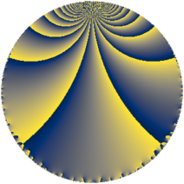# Properties

 Label 56.3.oLevel $56$ Weight $3$ Character orbit 56.o Rep. character $\chi_{56}(17,\cdot)$ Character field $\Q(\zeta_{6})$ Dimension $8$ Newform subspaces $1$ Sturm bound $24$ Trace bound $0$

# Related objects

## Defining parameters

 Level: $$N$$ $$=$$ $$56 = 2^{3} \cdot 7$$ Weight: $$k$$ $$=$$ $$3$$ Character orbit: $$[\chi]$$ $$=$$ 56.o (of order $$6$$ and degree $$2$$) Character conductor: $$\operatorname{cond}(\chi)$$ $$=$$ $$7$$ Character field: $$\Q(\zeta_{6})$$ Newform subspaces: $$1$$ Sturm bound: $$24$$ Trace bound: $$0$$

## Dimensions

The following table gives the dimensions of various subspaces of $$M_{3}(56, [\chi])$$.

Total New Old
Modular forms 40 8 32
Cusp forms 24 8 16
Eisenstein series 16 0 16

## Trace form

 $$8 q - 4 q^{7} + 20 q^{9} + O(q^{10})$$ $$8 q - 4 q^{7} + 20 q^{9} + 4 q^{11} + 8 q^{15} - 24 q^{17} - 84 q^{19} - 52 q^{21} - 48 q^{23} + 24 q^{25} - 104 q^{29} + 156 q^{31} + 204 q^{33} + 156 q^{35} + 68 q^{37} - 52 q^{39} + 160 q^{43} - 276 q^{45} - 108 q^{47} - 80 q^{49} - 180 q^{51} - 28 q^{53} - 8 q^{57} + 120 q^{59} + 252 q^{61} + 76 q^{63} + 220 q^{65} - 56 q^{67} - 208 q^{71} - 156 q^{73} - 576 q^{75} - 240 q^{77} - 160 q^{79} + 96 q^{81} - 88 q^{85} + 348 q^{87} + 204 q^{89} + 120 q^{91} + 268 q^{93} + 216 q^{95} + 728 q^{99} + O(q^{100})$$

## Decomposition of $$S_{3}^{\mathrm{new}}(56, [\chi])$$ into newform subspaces

Label Dim $A$ Field CM Traces $q$-expansion
$a_{2}$ $a_{3}$ $a_{5}$ $a_{7}$
56.3.o.a $8$ $1.526$ 8.0.$$\cdots$$.2 None $$0$$ $$0$$ $$0$$ $$-4$$ $$q-\beta _{5}q^{3}+\beta _{4}q^{5}+(1-3\beta _{1}-\beta _{2}+\beta _{3}+\cdots)q^{7}+\cdots$$

## Decomposition of $$S_{3}^{\mathrm{old}}(56, [\chi])$$ into lower level spaces

$$S_{3}^{\mathrm{old}}(56, [\chi]) \cong$$ $$S_{3}^{\mathrm{new}}(14, [\chi])$$$$^{\oplus 3}$$$$\oplus$$$$S_{3}^{\mathrm{new}}(28, [\chi])$$$$^{\oplus 2}$$